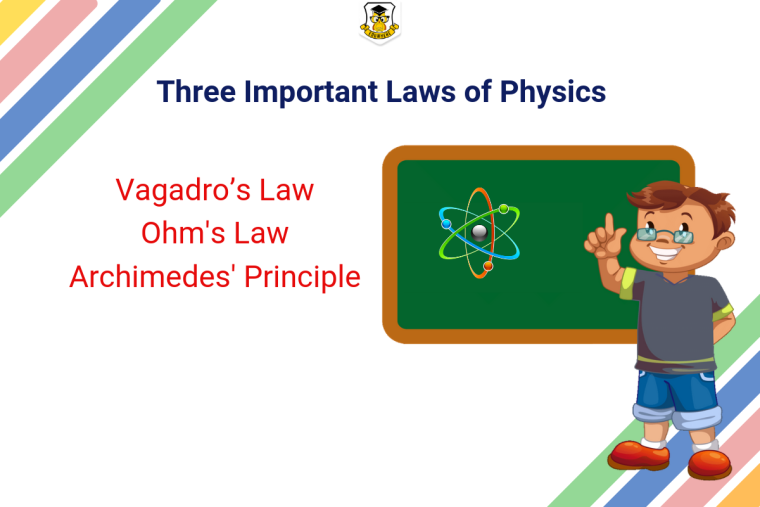## Eduwhere's Concept: 3 Important Laws of PhysicsHere are 3 important laws of Physics you should know for your upcoming examination:

1.Archimedes' Principle

The principle was discovered in 3rd century B.C. by the Greek mathematician. Archimedes. It states that when a body is partially or totally immersed in a fluid, it experiences an upward thrust equal to the weight of the fluid displaced by it that i.e. its apparent loss of weight is equal to the weight of liquid displaced.

Avagardro's law states that equal volumes of all gases under the same conditions of temperature and pressure contain an equal number of molecules. In 1811, this law was discovered by an Italian Scientist Anedeos Avagadro.

V ∝ n or V/n = k

Where “V” is the volume of the gas, “n” is the amount of the gas (number of moles of the gas) and “k” is a constant for a given pressure and temperature.

3. Ohm's Law

It states that the current passing through a conductor between two points is directly proportional to the potential difference across the two points provided the physical state and temperature etc. of the conductor does not change.

V = I R where V is the potential difference between two points which include a resistance R.

I is the current flowing through the resistance. For biological work, it is often preferable to use the conductance, g = 1/R; In this form Ohm's Law is:

I = g V

Visit Eduwhere to prepare for your upcoming competitive exams with the best online mock test series.

1

1399